M328K First Midterm Solutions, February 21, 2003

1. Using induction, prove the formula: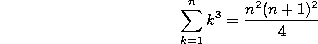We prove this by induction. The formula is true for the base case n=1, since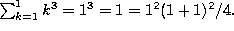Now for the inductive step. We assume the formula is true for n=m-1 and show it works for n=m. If it is true for n=m-1, then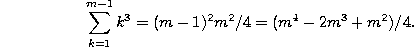But then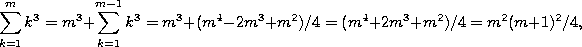which is the formula we needed to show.

2. As you know, the Fibonacci numbers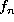are defined by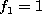,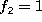and, for n>2,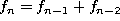. Give a rigorous proof of the assertion: ``is divisible by 3 if and only if n is divisible by 4.'' [Hint: Before writing down your proof, you may want to first determine which Fibonacci numbers are congruent to 1 (mod 3), which are congruent to 2 (mod 3), and which are divisible by 3. I'm sure you'll see the patters quickly enough.]

I claim that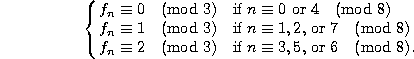If we can prove this, then the original assertion follows, since being divisible by 4 is the same as being congruent to 0 or 4 modulo 8.

We prove the claim by (generalized) induction. It is true for the base cases n=1 and n=2, since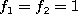. Now, for m;SPMgt;2, suppose that it is true for all values of n up to m-1. We must show it is true for n=m. There are 8 cases to check:

If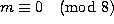, then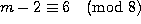and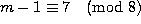, so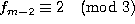and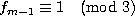, so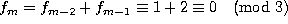.

If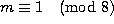, then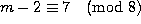and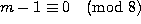, so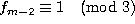and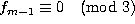, so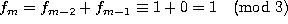.

Similarly, if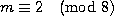, then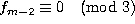and, so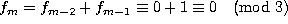.

If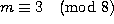, thenand, so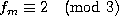.

If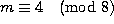, thenand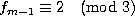, so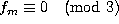.

If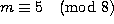, thenand, so.

If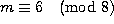, thenand, so.

If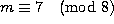, thenand, so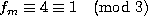, and then we're done.

3. Greatest common factors:

a) Find the greatest common factor of 66 and 52.

By the Euclidean algorithm: 66 = 1(52) + 14; 52 = 3(14) + 10; 14 = 1(10)+4; 10 = 2(4) + 2; 4 = 2(2). So (66,52)=2.

b) Write this number explicitly as a linear combination of 66 and 52. For instance, if (66,52) were equal to 24 (which it obviously isn't!), you might write ``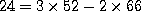''.

Working through the algorithm, we have 14 = 66 - 52; 10 = 52 - 3(14) = 4(52) - 3(66); 4 = 14 - 10 = 4(66) - 5(52); and finally our answer: 2 = 10 - 2(4) = 14(52) - 11(66).

c) What is the least common multiple of 66 and 52?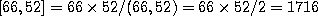.

4. Congruences, Diophantine equations and the Chinese Remainder Theorem.

a) Find all integer solutions to the equation 25 x + 38 y = 1.

By inspection, or by the Euclidean algorithm, you can see that -3(25) + 2(38) = -75 + 76 = 1. Thus ALL integer solutions are of the form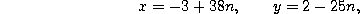where n is an arbitrary integer.

b) Find a solution to the equation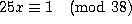.

This is the same question, and the answer is x=-3 (or x=35, or any number that is congruent to -3 modulo 38).

c) Find a solution to the equation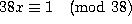.

There was a typo in the question.  It should have read "mod 25", not "mod 28".  As written, there is no answer, since the left hand side is always congruent to 0 (mod 38).  As intended, the answer is x=2 (or anything congruent to 2 modulo 25).

d) Find a positive solution to the congruences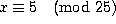,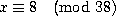.

Since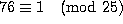and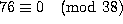, and since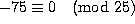and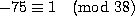, x=5(76)+8(-75) = -220 is congruent to 5 modulo 25 and 8 modulo 38. However, we wanted a POSITIVE solution, so we add an arbitrary multiple of (25)(38)=950. The smallest solution is -220+950 = 730.